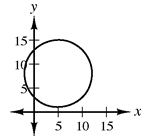### Home > CCA2 > Chapter 2 > Lesson 2.2.4 > Problem2-148

2-148.

Consider the equation $(x-5)^2+(y-8)^2=49$.

1. What can you tell about the graph just by looking at the equation?

Notice that both $x$ and $y$ are squared.

This is a circle with center $(5,8)$ and radius $7$.

2. Sketch a graph of $(x-5)^2+(y-8)^2=49$.